#### Murthy’s PPS Method

Murthy’s method (METHOD=PPS_MURTHY) selects two units from each stratum, with probability proportional to size and without replacement. The selection probability for unit i in stratum h equals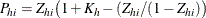where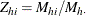and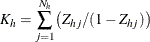Murthy’s algorithm first selects a unit with probability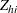. Then a second unit is selected from the remaining units with probability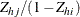, where unit i is the first unit selected. The joint selection probability for units i and j in stratum h equals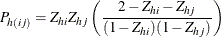See Cochran (1977, pp. 263–265) and Murthy (1957) for details.